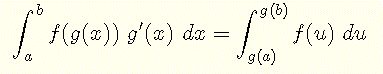#Free Mathematics Tutorials# Integration by Substitution

Tutorials with examples and detailed solutions and exercises with answers on how to use the powerful technique of integration by substitution to find integrals.

## Review Integration by Substitution

The method of integration by substitution may be used to easily compute complex integrals. Let us examine an integral of the formLet us make the substitution u = g(x), hence du/dx = g'(x) and du = g'(x) dx
With the above substitution, the given integral is given byIn what follows C is a constant of integration which is added in the final result.

## Examples

### Example 1

Evaluate the integral
sin(a x + b) dx

Solution to Example 1:
Let u = a x + b which gives du/dx = a or dx = (1/a) du. The substitution helps in computing the integral as follows
sin(a x + b) dx
= (1/a) sin(u) du
= (1/a) (-cos(u)) + C
= - (1/a) cos(a x + b) + C

### Example 2

Evaluate the integral
e3x - 2 dx

Solution to Example 2:
Let u = 3x - 2 which gives du/dx = 3 or dx = (1/3) du. Hence
e3x - 2 dx
= eu (1/3) du
= (1/3) eu
= (1/3) e3x - 2 + C

### Example 3

Evaluate the integral
x (2x2 + 5)4 dx

Solution to Example 3:
Let u = 2x2 + 5 which gives du/dx = 4x, du = 4x dx, (1/4) du = x dx . The substitution gives
x (2x2 + 5)4 dx
= (1/4) (u)4 du
= (1/4) (1/5) u5
= (1/20) (2x2 + 5)5 + C

### Example 4

Evaluate the integral
x √(2x + 1) dx

Solution to Example 4:
Let u = 2x + 1 which gives du/dx = 2 and dx = (1/2) du. Solve u = 2x + 1 for x to obtain x = (1/2)(u - 1). The substitution gives
x √(2x + 1) dx
= (1/2)(u - 1)(u)1/2 (1/2) du
= (1/4) (u3/2 - u1/2) du
= (1/4)( (2/5)u5/2 - (2/3)u3/2 )
= ( (2x + 1)3/2(3x - 1) ) / 15 + C

### Example 5

Evaluate the integral
(x - 5)-4 dx

Solution to Example 5:
Let u = x - 5 which gives du/dx = 1. Substituting into the given integral, we obtain
(x - 5)-4 dx
= u-4 du
= (-1/3)u-3
= (-1/3)(x - 5)-3 + C

### Example 6

Evaluate the integral
-x ex2 + 2 dx

Solution to Example 6:
Let u = x2 + 2 which gives du/dx = 2x and (1/2) du = x dx. A substitution into the given the integral gives
-x ex2 + 2 dx
= - eu (1/2) du
= - (1/2) eu du
= - (1/2) eu
= - (1/2) ex2 + 2 + C

### Example 7

Evaluate the integral
cos(x) sin4(x) dx

Solution to Example 7:
Let u = sin(x) which gives du/dx = cos(x) or cos(x) dx = du. Substitute into the integral to obtain
cos(x) sin4(x) dx = u4 du
= (1/5) u5
= (1/5) sin5(x) + C

### Example 8

Evaluate the integral
(3x / (4x + 1)) dx

Solution to Example 8:
Let u = 4x + 1 which gives du/dx = 4 or dx = (1/4) du. Solve u = 4x + 1 for x to obtain x = (1/4)(u - 1). Substitute to obtain
(3x / (4x + 1)) dx
= 3 (1/4) ( (u - 1) / u ) (1/4) du
= (3/16)(u - 1) / u du
= (3/16)(1 - 1/u) du
= (3/16)(u - ln|u|)
= (3/16)(4x + 1 - ln|4x + 1|) + C

### Example 9

Evaluate the integral
(x / √(x - 2)) dx

Solution to Example 9:
Let u = x - 2 which gives du/dx = 1, dx = du and x = u + 2. Substitution
(x / √(x - 2)) dx
= (u+2) / √u) dx
= (u1/2 + 2u-1/2) dx
= (2/3)u3/2 + 2 * 2 u1/2
= (2/3)(x - 2)3/2 + 4(x - 2)1/2 + C

### Example 10

Evaluate the integral
(x + 2)3(x + 4)2 dx

Solution to Example 10:
Let u = x + 2 which gives du/dx = 1, dx = du and also x = u - 2. Using the above substitution we obtain
(x + 2)3(x + 4)2 dx
= u3(u + 2)2 du
= (u5 + 4u4 + 4u3)du
= (1/6) u6 + (4/5)u5 + u4
= (1/6)(x + 2)6 + (4/5)(x + 2)5 + (x + 2)4 + C

### Example 11

Evaluate the integral
( (2x + 3) / (x2 + 3x + 1) ) dx

Solution to Example 11:
Let u = x2 + 3x + 1 which gives du/dx = 2x + 3 or (2x + 3) dx = du. The substitution helps in computing the integral as follows
( 1 / u ) du
= ln|u|
= ln |x2 + 3x + 1| + C

## Exercises

Use the table of integrals and the method of integration by parts to find the integrals below. [Note that you may need to use the method of integration by parts more than once].
1.
cos(3x - 2) dx
2.
e 4x - 7 dx
3.
x(4x 2 + 5) 4 dx
4.
1 / (x + 3) 3 dx

1. (1/3) sin(3x - 2)
2. (1/4) e
4x - 7 + C
3. (1/40) (4x
2 + 5) 5 + C
4. (-1/2) 1 / (x + 3)
2 + C